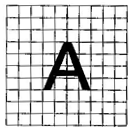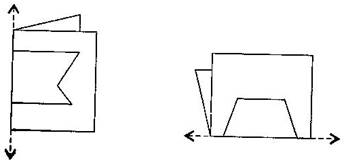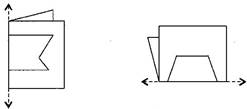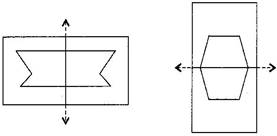### Symmetry-Solutions Ex-13.2

CBSE Class –VI Mathematics
NCERT Solutions
Chaper 13 Symmetry (Ex. 13.2)

Question 1. Find the number of lines of symmetry for each of the following shapes: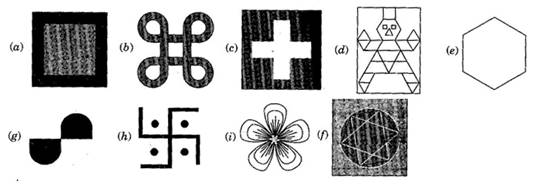(a) 4, (b) 4, (c) 4, (d) 1, (e) 6, (f) 4, (g) 0, (h) 0, (i) 3
Question 2. Copy the triangle in each of the following figures, on squared paper. In each case, draw the line(s) of symmetry. If any and identity the type of triangle. (Some of you may like to trace the figures and try paper-folding first!)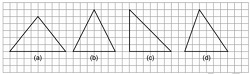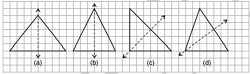(a) ${l}_{1}$ is the line of symmetry.(Isosceles triangle)
(b) ${l}_{1}$ is the line of symmetry. (equilateral triangle. the line of symmetries are 3)
(c) ${l}_{1}$ is the line of symmetry.(Right angled triangle)
(d) No line of symmetry. (Scalene triangle)

Question 3.Complete the following table:
 Shape Rough figure No. of lines of symmetry Equilateral triangle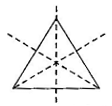3 Square Rectangle Isosceles triangle Rhombus Circle

 Shape Rough figure No. of lines of symmetry Equilateral triangle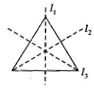3 Square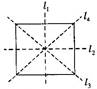4 Rectangle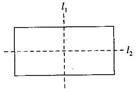2 Isosceles triangle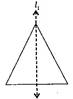1 Rhombus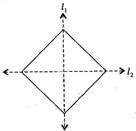2 Circle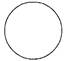Infinite (Countless)

Question 4.Can you draw a triangle which has:
(a) exactly one line of symmetry?
(b) exactly two lines of symmetry?
(c) exactly three lines of symmetry?
(d) no lines of symmetry?
Sketch a rough figure in each case.
(a) Yes, Isosceles triangle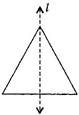(b) No such triangle cannot be formed.
(c) Yes, Equilateral triangle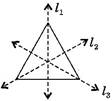(d) Yes, Scalene triangle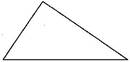Question 5.On a squared paper, sketch the following:
(a) A triangle with a horizontal line of symmetry but no vertical line of symmetry.
(b) A quadrilateral with both horizontal and vertical lines of symmetry.
(c) A quadrilateral with a horizontal line of symmetry but no vertical line of symmetry.
(d) A hexagon with exactly with two lines of symmetry.
(e) A hexagon with six lines of symmetry.
(Hint: It will be helpful if you first draw the lines of symmetry and then complete the figures)
(a)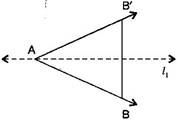(b)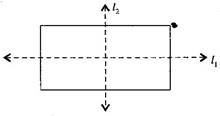(c)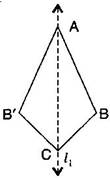(d)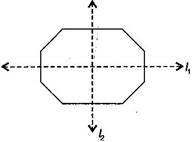(e)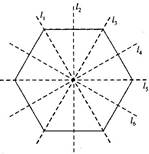Question 6.Trace each figure and draw the lines of symmetry, if any: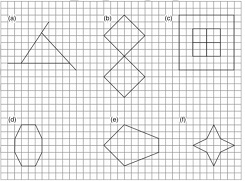Answer: The line(s) of symmetry of the given figures are shown as under by dotted lines :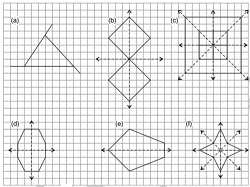Question 7.Consider the letters of English alphabets A to Z. List the letters which have:
(a) vertical lines of symmetry (like A)
(b) horizontal lines of symmetry (like B)
(c) no lines of symmetry (like Q)